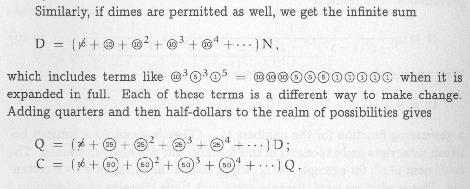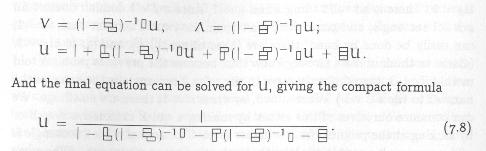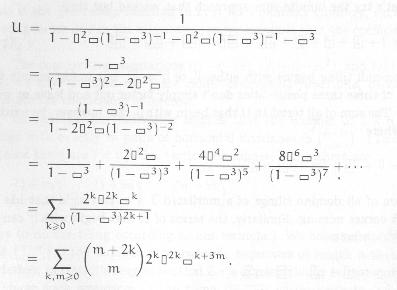# Guidelines for Graphics in MathML 2

## W3C Note 01 July 2003

This version:
http://www.w3.org/Math/Documents/Notes/aphics/graphics.xml
http://www.w3.org/Math/Documents/Notes/graphics.xml
Previous version:
none
Editors:
Michael Kohlhase, Carnegie Mellon University <kohlhase+@cs.cmu.edu>
David Carlisle, NAG <davidc@nag.co.uk>

## Abstract

In this note, we will develop guidelines for the integration of graphics in MathML2 [MathML2]. We will frame much of the discussion of the extended use of MathML in SVG and SVG in MathML, and discuss current tool support. We will discuss some use cases for mathematics with diagrams. We will close with some general remarks about mixing namespaces in XML.

## 1 Introduction

A distinguishing feature of mathematics is the use of a complex and highly evolved system of two-dimensional symbolic notations. The MathML [MathML2] format is the World Wide Web Consortitum's (W3C) answer to the problem of the problem of communicating these notations on the web.

However, MathML does not cover all mathematical practice; due to the inherent complexity of the material, mathematicicans also make use of various forms of diagrams to visualize their ideas and the objects in question. For diagrams, the W3C provides the Scalable Vector Graphics (SVG) format [SVG1.1].

In isolation, both MathML and SVG are very successful, they cover the respective practice, and are supported in numerous browsers, printer drivers, and authoring tools. The W3C has put out a joint DTD and Schema [XHTML-MathML-SVG] for a modular integration of MathML and SVG into XHTML.

Unfortuntaely, this is not enough to cover mathematical practice. Since the diagrams are about mathematical objects, they can contain mathematical formulae, and since diagrams are sometimes used to communicate mathemtatical objects they can occur inside mathematical formulae. This practice, requires a mixing of MathML and SVG, which is not explicitly supported by the existing recommendations. Moreover, this extended use of MathML and SVG is only marginally covered by existing implementations.

Currently, the only document markup system that can combine mathematics with diagrams is the TeX/LaTeX suite of programs. In this note we will develop guidelines for the extended use of mixing MathML and SVG, and discuss current tool support based practical cases where graphics and mathematical notation are mixed. These will serve as the basis for the discussion in the next section. We can distinguish three cases:

As we will see, the first two can be solved by interpretations of the current recommentations, while the third one is problematic. We will close with some general remarks about mixing namespaces in XML.

## 2 Mathematical Formulae in Diagrams

### 2.2 Guidelines

The XHTML + MathML + SVG Profile [XHTML-MathML-SVG] allows to embed MathML into SVG inside the `foreignobject` element. Consider the following example (adapted for MathML content from the SVG 1.1 recommendation [SVG1.1]).

```<?xml version="1.0" standalone="yes"?>
<svg width="4in" height="3in" version="1.1" xmlns = 'http://www.w3.org/2000/svg'>
<desc>This example uses the 'switch' element to provide a
fallback graphical representation of an paragraph, if
MathML is not supported.</desc>
<!-- The 'switch' element will process the first child element
whose testing attributes evaluate to true.-->
<switch>

<!-- Process the embedded MathML if the requiredExtensions attribute
evaluates to true (i.e., the user agent supports MathML embedded within SVG). -->
<foreignObject width="100" height="50"
requiredExtensions="xmlns="http://www.w3.org/1998/Math/MathML">
<!-- MathML content goes here -->
<math xmlns="http://www.w3.org/1998/Math/MathML>
<mfrac>
<mn> 1 </mn>
<mrow>
<msup><mi> x </mi><mn> 3 </mn></msup>
<mo> + </mo>
<mfrac><mi> x </mi><mn> 3 </mn></mfrac>
</mrow>
</mfrac>
[/itex]
</foreignObject>

<!-- Else, process the following alternate SVG. -->
<text font-size="10" font-family="italic">
<tspan x="10" y="10">1/(x^3+(x/3))</tspan>
</text>
</switch>
</svg>```

Note that the `width` and `height` attributes on the `foreignObject` are required, which may pose a problem for embedded MathML objects, which scale by different mechanisms than diagrams (glyphs re-sizing does not always conserve aspect ratio),

### 2.3 Implementation Issues

 Editorial note: MiKo Need to talk about implementations should mention Lavirotte's MathML to SVG converter, David's stylesheet approach, etc. AMAYA can already do it, maybe a MathML+SVG-enabled Mozilla as well (I have not found one).

## 3 Diagrams as Sub-Formulae

In this section discuss the case of diagrams or images inside of mathematical formulae. We will looke at some use cases and the give guidelines to accomodate diagrams and images in MathML representations.

### 3.1 Use Cases

Let us look at some example of images or diagrams inside mathematical formulae. Since MathML supplies the `mglyph` element, the question we have to ask ourselves in all of these cases, whether the diagram is not really a specialized glyph

Even though the use of diagrams or images inside of mathematical formulae is relatively rare in published mathematics (an exception seems to be slide presentations or other educational material), such cases do exist, e.g. in Graham, Knuth, Patashnik: Concrete Mathematics, where the authors use the first formula below to talk about the probabilities of certain events involing dice and the second one for theories about the number of ways to pay a certain amount using a variety of coins.In these example, it is somewhat plausible to think that the dice (after all the dice are present Unicode as characters 2680 (1) to 2685 (6). But the coins are not, they could however be integrated into MathML using the `mglyph` element; The MathML specification has this example about braid group notation.

Later in the book Graham, Knuth, Patashnik talk about tiling rectangles with dominoes, and use the following formulaeHere the assumption that the complex configurations of dominos are glyphs becomes more implausible, after all, there are infinitely many of the. Consider this formula, to see that the math done with these kinds of diagrams is not restricted to trivial formulaeFinally, they use the following formula to reason about graphs.### 3.2 Guidelines

The MathML2 specification provides the `semantics` element for

"The `semantics` element is designed to group together various sources of information related to a particular mathematical object. For example, it is often used to provide both a content representation of the object and a presentation."

Clearly, the graphical representation of a mathematical object is a "presentation" of the element, so we can use the `semantics` element as in the following example:

```<semantics>
<csymbol definitionURL="elementary-stats.html#dice-4up"/>
<annotation-xml encoding="SVG1.1">
<svg xmlns="http://www.w3.org/2000/svg" height="137px">
<rect stroke="black" fill="none" rx="5px" width="40px" height="40px"/>
<circle stroke="black" fill="black" cy="30px" cx="30px" r="5px"/>
<circle stroke="black" fill="black" cy="30px" cx="10px" r="5px"/>
<circle stroke="black" fill="black" cy="20px" cx="20px" r="5px"/>
<circle stroke="black" fill="black" cy="10px" cx="30px" r="5px"/>
<circle stroke="black" fill="black" cy="10px" cx="10px" r="5px"/>
</svg>
</annotation-xml>
<annotation encoding="image/jpeg"> copy dice4.jpg here </annotation>
<annotation encoding="image/gif" other="dice.gif"/>
</semantics>```

The first child of the `semantics` element is the MathML object whith which the additional information is associated, it can be a presentation- or content MathML representation. This child is mandatory in a `semantics` element, it can be seen as a fallback representation in MathML, if the MathML application processing the `semantics` element cannot process any of the `annotation` and `annotation-xml` children. In our case, we have chosen to represent the dice as a `csymbol` element, another choice would have been to give an alternate text represented in a `mtext` element.

### 3.3 Implementation Issues

 Editorial note: MiKo Need to talk about implementations. Again, a MathML to SVG converter can handle this (potentially). What about AMAYA, Mozilla? We need a worked-out example for this.

## 5 Conclusions

We have discussed some use-cases for an integration of the images and and MathML specifications. Simple mathematical diagrams can already be represented in current SVG+MathML applications, but for many relevant classes of mathematical diagrams, the state of the art is not sufficient for mathematical practice. Currently, the only document markup system that can combine mathematics with diagrams is the TeX/LaTeX suite of programs. To make MathML (and the W3C languages) a viable alternative for main-stream technical publications, we need to develop the mixture of MathML and SVG further, alleviating the problems discussed in this note.

Note that these are only an instance of the more general problem of mixing namespaces in general. The hierarchical mixing cases discussed in sections 2 Mathematical Formulae in Diagrams and 3 Diagrams as Sub-Formulae are relatively simple, since the embeddings respect bounding boxes. Presentation agents only need to negotiate box sizes, alignment, and flow of control. Moreover, nesting depths for namespaces with depth greater than one (e.g. SVG in MathML in SVG) seem very rare. But already the very natural mixed case discussed in 4 Non-Hierarchical Mixing of Formulae and Graphics, break this hierarchical black-box model. In particular, prsentation agents would have to negotiate inner coordinates, etc.

 Editorial note: MiKo Talk about general Problems of mixing Namespaces in XML

## A Listings for the Examples

### A.1 The commutative diagram

 Editorial note: MiKo adapt to the SVG guidelines
```<svg xmlns="http://www.w3.org/2000/svg" width="10em" height="5.6em"
style="stroke:black font-family:serif">
<defs><polygon id="ArrowHead" points="0,0 4,0 2,6" transform="translate(-2,-6)"/></defs>
<text x="0" y="1em">
<math xmlns="http://www.w3.org/1998/Math/MathML"><msub><mi>C</mi><mi>&theta;</mi></msub>[/itex]
</text>
<text x="2em" y="1em">
<math xmlns="http://www.w3.org/1998/Math/MathML">
<msub><mo>&vdash;</mo><mrow><mi>R</mi><mi>C</mi></mrow></msub>
[/itex]
</text>
<text x="4em" y="1em">
<math xmlns="http://www.w3.org/1998/Math/MathML">
<msubsup><mi>C</mi><mi>&theta;</mi><mo>&prime;&prime;</mo></msubsup>
[/itex]<
/text>
<text x="6em" y="1em">
<math xmlns="http://www.w3.org/1998/Math/MathML">
<msub><mo>&vdash;</mo><mrow><mi>R</mi><mi>C</mi></mrow></msub>
[/itex]
</text>
<text x="8em" y="1em">
<math xmlns="http://www.w3.org/1998/Math/MathML">
<msubsup><mi>C</mi><mi>&theta;</mi><mo>&prime;</mo></msubsup>
[/itex]
</text>
<text x=".3em" y="3em" text-anchor="end">
<math xmlns="http://www.w3.org/1998/Math/MathML"><mi>&omega;</mi>[/itex]
</text>
<text x=".7em" y="3em">
<math xmlns="http://www.w3.org/1998/Math/MathML"><mi>&theta;</mi>[/itex]
</text>
<text x="4.3em" y="3em" text-anchor="end">
<math xmlns="http://www.w3.org/1998/Math/MathML">
<msup><mi>&omega;</mi><mo>&prime;&prime;</mo></msup>
[/itex]
</text>
<text x="4.7em" y="3em">
<math xmlns="http://www.w3.org/1998/Math/MathML">
<msup><mi>&theta;</mi><mo>&prime;&prime;</mo></msup>
[/itex]
</text>
<text x="8.3em" y="3em" text-anchor="end">
<math xmlns="http://www.w3.org/1998/Math/MathML">
<msup><mi>&omega;</mi><mi>&prime;</mi></msup>
[/itex]
</text>
<text x="8.7em" y="3em">
<math xmlns="http://www.w3.org/1998/Math/MathML">
<msup><mi>&theta;</mi><mi>&prime;</mi></msup>
[/itex]
</text>
<text x="0" y="5em">
<math xmlns="http://www.w3.org/1998/Math/MathML"><mi>C</mi>[/itex]
</text>
<text x="2em" y="5em">
<math xmlns="http://www.w3.org/1998/Math/MathML">
<msub><mo>&vdash;</mo><mi>p</mi></msub>
[/itex]
</text>
<text x="4em" y="5em">
<math xmlns="http://www.w3.org/1998/Math/MathML">
<msup><mi>C</mi><mo>&prime;&prime;</mo></msup>
[/itex]
</text>
<text x="6em" y="5em">
<math xmlns="http://www.w3.org/1998/Math/MathML">
<msub><mo>&vdash;</mo><mrow><mi>I</mi><mi>H</mi></mrow></msub>
[/itex]
</text>
<text x="8em" y="5em">
<math xmlns="http://www.w3.org/1998/Math/MathML">
<msup><mi>C</mi><mi>&prime;</mi></msup>
[/itex]
</text>
<line x1=".5em" y1="1.4em" x2=".5em" y2="4.1em"/>
<line x1="4.5em" y1="1.4em" x2="4.5em" y2="4.1em"/>
<line x1="8.5em" y1="1.4em" x2="8.5em" y2="4.1em"/>
</svg>
```

### A.2 Ebellished Formulae

Let us now consider markup for the $\lambda$ example in 4.1 Use Cases here. Vincent Quint has proposed the following mixed SVG/MathML markup:

```<svg xmlns="http://www.w3.org/2000/svg" width="15.2em"
height="45px" style="stroke:black font-family:serif">
<text y="22px" x="0">
<math xmlns="http://www.w3.org/1998/Math/MathML">
<mrow>
<mo fence="true">(</mo>
<mi>&lambda;</mi>
<mi>x</mi>
<mo>.</mo>
<mi>f</mi>
<mrow>
<mo fence="true">(</mo>
<mi>x</mi>
<mo>,</mo>
<mi>f</mi>
<mrow><mo fence="true">(</mo><mi>x</mi><mo fence="true">)</mo></mrow>
<mo fence="true">)</mo>
</mrow>
<mo fence="true">)</mo>
</mrow>
<munder>
<mrow>
<mi>g</mi>
<mrow><mo fence="true">(</mo><mi>a</mi><mo fence="true">)</mo></mrow>
</mrow>
<mo>&UnderBrace;</mo>
</munder>
<mo>&RightArrow;</mo>
<mi>&beta;</mi>
<mspace width="0.3em"/>
<mi>f</mi>
<mrow>
<mo fence="true">(</mo>
<mi>g</mi>
<mrow><mo fence="true">(</mo><mi>a</mi><mo fence="true">)</mo></mrow>
<mo>,</mo>
<mi>f</mi>
<mrow>
<mo fence="true">(</mo>
<mi>g</mi>
<mrow><mo fence="true">(</mo><mi>a</mi><mo fence="true">)</mo></mrow>
<mo fence="true">)</mo>
</mrow>
<mo fence="true">)</mo>
</mrow>
[/itex]
</text>
<path fill="none" d="M 15,13 C 14,11 20,6 24,7 C 27,7 34,12 34,14"
style="stroke: #BEBEBE"/>
<path fill="none"
d="M 15,13 C 12,9 20,3 24,2 C 29,0 39,2 44,4 C 46,5 54,9 54,12"
style="stroke: #BEBEBE"/>
<path fill="none"
d="M 53,23 C 51,25 54,31 57,33 C 63,37 75,37 82,36 C 84,35 89,32 89,31"/>
<path fill="none"
d="M 32,23 C 29,26 36,35 41,38 C 49,43 67,44 77,42 C 81,40 89,35 89,31"/>
</svg>```

which gives the correct presentation. Note that since this is modeled as "MathML in SVG", the SVG application can plot the arcs represented in the `path` elements over the formula. Note that the `path` elements are given in absolute coordinates; since mathematical formulae scale by different mechanisms than diagrams (glyphs re-sizing does not always conserve aspect ratio), the visual connection need not be invariant under resizing.

However, given that the example is really a mathematical formula with embellishments, a "SVG in MathML" representation would have been more appropriate semantically. We have speculated about a possible representation with a view towards TeX/LaTeX (and in particular the `pstricks` package. This representation annotates a MathML formula (we have only represented the left hand side for brevity) with the arcs in a `semantics` element. In contrast to the representation above, we have not used current SVG `path` elements with absolute coordinates, but we have postulated a `svg:connection` (such functionality does not exist in SVG 1.1) with a suitable `shape` attribute to obtain the effect. In contrast to the example in section A.1 The commutative diagram, where we referenced to `ID`-type attributes on SVG attributes, we rely on `ID` attributes of MathML elements. This has the effect for an SVG+MathML application that the MathML rendering cannot be included as a black box any more, but must advertise the bounding boxes of labeled sub-expressions to the calling SVG application.

```<math style="display">
<semantics>
<mrow>
<mo fence="true">(</mo>
<mo>&lambda;</mo>
<mi id="s1">x</mi>
<mo>.</mo>
<mrow>
<mo>f</mo>
<mrow>
<mo fence="true">(</mo>
<mi id="t1">x</mi>
<mo separator="true">,</mo>
<mi id="t2">x</mi>
<mo fence="true">)</mo>
</mrow>
</mrow>
<mo fence="true">)</mo>
<mrow id="s2">
<mo>g</mo>
<mrow><mo fence="true">(</mo><mi>a</mi><mo fence="true">)</mo></mrow>
</mrow>
</mrow>
<annotation-xml encoding="image/svg" definitionURL="embellishments">
<svg:connection from="s1" to="t1" style="dotted" shape="arc"/>
<svg:connection from="s1" to="t2" style="dotted" shape="arc"/>
<svg:connection from="s2" to="t1" shape="arc"/>
<svg:connection from="s2" to="t2" shape="arc"/>
</annotation-xml>
</semantics>
[/itex]```

Note that a presentation agent would have to report the locations of the MathML elements with the `id` attributes referred to by the `svg:connection` elements.

## B Bibliography

XHTML-MathML-SVG
Masayasu Ishikawa, ed., An XHTML + MathML + SVG Profile, W3C Working Draft
SVG1.1
Dean Jackson, Jon Ferraiolo, Jun Fujisawa, eds. Scalable Vector Graphics (SVG) 1.1 Specification, W3C Candidate Recommendation 30 April 2002
MathML2
David Carlisle, Patrick Ion, Robert Miner, Nico Poppelier, Mathematical Markup Language (MathML) Version 2.0 W3C Recommendation 21 February 2001